# 轻松实现坐标转换文档选项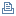打印本页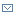将此页作为电子邮件发送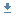样例代码

Sami Salkosuo (mailto:sami.salkosuo@fi.ibm.com?subject=轻松实现坐标转换), 应用程序架构师, IBM

2007 年 9 月 07 日UTM 坐标系统使用基于网格的方法表示坐标。UTM 系统将地球分为 60 个区，每个区基于横轴墨卡托投影。绘图法中的地图投影方法可以在平面中表示一个两维的曲面，例如一个标准地图。图 2 展示了一个横轴墨卡托投影：UTM 经度区范围为 1 到 60；其中 58 个区的东西跨度为 6°（稍后详细讨论另外两个区）。经度区涵盖了地球中纬度范围从 80°S 到 84°N 之间的所有区域。UTM 坐标的表示格式为：经度区纬度区以东以北，其中以东 表示从经度区的中心子午线的投影距离，而以北 表示距离赤道的投影距离。这个两个值的单位均为米。举例来说，使用 UTM 表示经/纬度坐标 61.44，25.40 的结果就是 35 V 414668 6812844；而经/纬度坐标 -47.04，-73.48 的表示结果为 18 G 615471 4789269。

MGRS 是北约（NATO）军事组织使用的标准坐标系统。MGRS 以 UTM 为基础并进一步将每个区划分为 100 km × 100 km 的小方块。这些方块使用两个相连的字母标识：第一个字母表示经度区的东西位置，而第二个字母表示南北位置。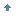回页首

DD 和 DMS 坐标格式之间的转换非常简单。下面给出了 DD 到 DMS 的转换公式：

 DD: dd.ff DMS: dd mm ss dd=dd mm.gg=60*ff ss=60*gg 

 lat dd=61 lat mm.gg=60*0.44=26.4 lat ss=60*0.4=24 

 lon dd=25 lon mm.gg=60*0.40=24.0 lon ss=60*0.0=0 

 DD: dd.ff DMS: dd mm ss dd.ff=dd + mm/60 + ss/3600 

 lat dd.ff= - (47 + 2/60 + 24/3600 )=-47.04 lon dd.ff= - (73 + 28/60 + 48/3600)=-73.48回页首

CoordinateConversion 的源代码包含大约 750 行代码，因此本文没有全部显示。以下小节描述了有关方法，本文的 下载 小节中附带了完整的源代码。

CoordinateConversion

CoordinateConversion 是主类，它被实例化为在需要是执行坐标转换。清单 1 展示了相关的公共方法，以及 CoordinateConversion 类中包含的私有内部类：

  public class CoordinateConversion { public CoordinateConversion() { } public double[] utm2LatLon(String UTM) { UTM2LatLon c = new UTM2LatLon(); return c.convertUTMToLatLong(UTM); } public String latLon2UTM(double latitude, double longitude) { LatLon2UTM c = new LatLon2UTM(); return c.convertLatLonToUTM(latitude, longitude); } //..implementation omitted private class LatLon2UTM { public String convertLatLonToUTM(double latitude, double longitude) { //..implementation omitted } //..implementation omitted } private class LatLon2MGRUTM extends LatLon2UTM { public String convertLatLonToMGRUTM(double latitude, double longitude) { //..implementation omitted } //..implementation omitted } private class MGRUTM2LatLon extends UTM2LatLon { public double[] convertMGRUTMToLatLong(String mgrutm) { //..implementation omitted } //..implementation omitted } private class UTM2LatLon { public double[] convertUTMToLatLong(String UTM) { //..implementation omitted } //..implementation omitted } private class Digraphs { //used to get digraphs when doing conversion between //lat/long and MGRS //..implementation omitted } private class LatZones { //include methods to determine latitude zones //..implementation omitted } 

  public String convertLatLonToUTM(double latitude, double longitude) { validate(latitude, longitude); String UTM = ""; setVariables(latitude, longitude); String longZone = getLongZone(longitude); LatZones latZones = new LatZones(); String latZone = latZones.getLatZone(latitude); double _easting = getEasting(); double _northing = getNorthing(latitude); UTM = longZone + " " + latZone + " " + ((int) _easting) + " "+ ((int) _northing); return UTM; } 

  protected void setVariables(double latitude, double longitude) { latitude = degreeToRadian(latitude); rho = equatorialRadius * (1 - e * e) / POW(1 - POW(e * SIN(latitude), 2), 3 / 2.0); nu = equatorialRadius / POW(1 - POW(e * SIN(latitude), 2), (1 / 2.0)); double var1; if (longitude < 0.0) { var1 = ((int) ((180 + longitude) / 6.0)) + 1; } else { var1 = ((int) (longitude / 6)) + 31; } double var2 = (6 * var1) - 183; double var3 = longitude - var2; p = var3 * 3600 / 10000; S = A0 * latitude - B0 * SIN(2 * latitude) + C0 * SIN(4 * latitude) - D0 * SIN(6 * latitude) + E0 * SIN(8 * latitude); K1 = S * k0; K2 = nu * SIN(latitude) * COS(latitude) * POW(sin1, 2) * k0 * (100000000) / 2; K3 = ((POW(sin1, 4) * nu * SIN(latitude) * Math.pow(COS(latitude), 3)) / 24) * (5 - POW(TAN(latitude), 2) + 9 * e1sq * POW(COS(latitude), 2) + 4 * POW(e1sq, 2) * POW(COS(latitude), 4)) * k0 * (10000000000000000L); K4 = nu * COS(latitude) * sin1 * k0 * 10000; K5 = POW(sin1 * COS(latitude), 3) * (nu / 6) * (1 - POW(TAN(latitude), 2) + e1sq * POW(COS(latitude), 2)) * k0 * 1000000000000L; A6 = (POW(p * sin1, 6) * nu * SIN(latitude) * POW(COS(latitude), 5) / 720) * (61 - 58 * POW(TAN(latitude), 2) + POW(TAN(latitude), 4) + 270 * e1sq * POW(COS(latitude), 2) - 330 * e1sq * POW(SIN(latitude), 2)) * k0 * (1E+24); } 

  protected String getLongZone(double longitude) { double longZone = 0; if (longitude < 0.0) { longZone = ((180.0 + longitude) / 6) + 1; } else { longZone = (longitude / 6) + 31; } String val = String.valueOf((int) longZone); if (val.length() == 1) { val = "0" + val; } return val; } 

getNorthing() 方法（清单 5）和 getEasting() 方法（清单 6）计算以北和以东的值。两种方法都使用 清单 3 中的 setVariables() 方法设置的变量。

  protected double getNorthing(double latitude) { double northing = K1 + K2 * p * p + K3 * POW(p, 4); if (latitude < 0.0) { northing = 10000000 + northing; } return northing; } 

  protected double getEasting() { return 500000 + (K4 * p + K5 * POW(p, 3)); } 

  ( 0.0000 0.0000 ) "31 N 166021 0" ( 0.1300 -0.2324 ) "30 N 808084 14385" (-45.6456 23.3545 ) "34 G 683473 4942631" (-12.7650 -33.8765 ) "25 L 404859 8588690" (-80.5434 -170.6540) "02 C 506346 1057742" ( 90.0000 177.0000) "60 Z 500000 9997964" (-90.0000 -177.0000) "01 A 500000 2035" ( 90.0000 3.0000 ) "31 Z 500000 9997964" ( 23.4578 -135.4545) "08 Q 453580 2594272" ( 77.3450 156.9876) "57 X 450793 8586116" (-89.3454 -48.9306 ) "22 A 502639 75072" 

UTM 坐标到经纬度坐标的转换要比相反的转换过程容易一些。同样，“The Universal Grids”（请参阅 参考资料）提供了转换公式。清单 8 展示了 convertUTMToLatLong() 方法的代码。该方法返回一个双数组，其中第一个元素（数组索引 ）表示纬度，而第二个元素（数组索引 ）表示经度。由于 UTM 字符串参数的精度为 1 米，因此经纬度坐标具有与之相同的精度。

  public double[] convertUTMToLatLong(String UTM) { double[] latlon = { 0.0, 0.0 }; String[] utm = UTM.split(" "); zone = Integer.parseInt(utm); String latZone = utm; easting = Double.parseDouble(utm); northing = Double.parseDouble(utm); String hemisphere = getHemisphere(latZone); double latitude = 0.0; double longitude = 0.0; if (hemisphere.equals("S")) { northing = 10000000 - northing; } setVariables(); latitude = 180 * (phi1 - fact1 * (fact2 + fact3 + fact4)) / Math.PI; if (zone > 0) { zoneCM = 6 * zone - 183.0; } else { zoneCM = 3.0; } longitude = zoneCM - _a3; if (hemisphere.equals("S")) { latitude = -latitude; } latlon = latitude; latlon = longitude; return latlon; } 

convertUTMToLatLong() 方法将传入的 UTM 字符串（格式为 34 G 683473 4942631）分解，并使用 getHemisphere() 方法确定字符串表示的位置所在的半球。这种确定非常简单：纬度区 ACDEFGHJKLM 位于南半球，而其余区位于北半球。

  protected void setVariables() { arc = northing / k0; mu = arc / (a * (1 - POW(e, 2) / 4.0 - 3 * POW(e, 4) / 64.0 - 5 * POW(e, 6) / 256.0)); ei = (1 - POW((1 - e * e), (1 / 2.0))) / (1 + POW((1 - e * e), (1 / 2.0))); ca = 3 * ei / 2 - 27 * POW(ei, 3) / 32.0; cb = 21 * POW(ei, 2) / 16 - 55 * POW(ei, 4) / 32; cc = 151 * POW(ei, 3) / 96; cd = 1097 * POW(ei, 4) / 512; phi1 = mu + ca * SIN(2 * mu) + cb * SIN(4 * mu) + cc * SIN(6 * mu) + cd * SIN(8 * mu); n0 = a / POW((1 - POW((e * SIN(phi1)), 2)), (1 / 2.0)); r0 = a * (1 - e * e) / POW((1 - POW((e * SIN(phi1)), 2)), (3 / 2.0)); fact1 = n0 * TAN(phi1) / r0; _a1 = 500000 - easting; dd0 = _a1 / (n0 * k0); fact2 = dd0 * dd0 / 2; t0 = POW(TAN(phi1), 2); Q0 = e1sq * POW(COS(phi1), 2); fact3 = (5 + 3 * t0 + 10 * Q0 - 4 * Q0 * Q0 - 9 * e1sq) * POW(dd0, 4) / 24; fact4 = (61 + 90 * t0 + 298 * Q0 + 45 * t0 * t0 - 252 * e1sq - 3 * Q0 * Q0) * POW(dd0, 6) / 720; lof1 = _a1 / (n0 * k0); lof2 = (1 + 2 * t0 + Q0) * POW(dd0, 3) / 6.0; lof3 = (5 - 2 * Q0 + 28 * t0 - 3 * POW(Q0, 2) + 8 * e1sq + 24 * POW(t0, 2)) * POW(dd0, 5) / 120; _a2 = (lof1 - lof2 + lof3) / COS(phi1); _a3 = _a2 * 180 / Math.PI; } 

setVariables() 使用以东和以北值设置所需的变量。这些都是类变量并且在 convertUTMToLatLong(String UTM) 方法中进行设置（参见 清单 8）。回页首回页首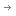关于下载方法的信息Sami Salkosuo 从 1999 年起一直在 IBM 工作。他是 Sun 认证的 Java 程序员，IBM 认证的 WebSphere Message Broker 解决方案开发人员，还是 IBM 认证的 WebSphere MQ 的解决方案设计师。

10-0209-2978
07-236648
12-29137
01-123563
06-11612
01-071358
08-13419
10-126万+
03-18137
04-201461
04-221007
07-061808
02-102万+
10-311053
07-02467
11-271308
07-238144点击重新获取扫码支付余额充值